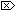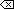The Open Group Base Specifications Issue 6
IEEE Std 1003.1-2001

#### NAME

y0, y1, yn - Bessel functions of the second kind

#### SYNOPSIS

```[XSI]#include <math.h> double y0(double x); double y1(double x); double yn(int n, double x);```

#### DESCRIPTION

The y0(), y1(), and yn() functions shall compute Bessel functions of x of the second kind of orders 0, 1, and n, respectively.

An application wishing to check for error situations should set errno to zero and call feclearexcept(FE_ALL_EXCEPT) before calling these functions. On return, if errno is non-zero or fetestexcept(FE_INVALID | FE_DIVBYZERO | FE_OVERFLOW | FE_UNDERFLOW) is non-zero, an error has occurred.

#### RETURN VALUE

Upon successful completion, these functions shall return the relevant Bessel value of x of the second kind.

If x is NaN, NaN shall be returned.

If the x argument to these functions is negative, -HUGE_VAL or NaN shall be returned, and a domain error may occur.

If x is 0.0, -HUGE_VAL shall be returned and a range error may occur.

If the correct result would cause underflow, 0.0 shall be returned and a range error may occur.

If the correct result would cause overflow, -HUGE_VAL or 0.0 shall be returned and a range error may occur.

#### ERRORS

These functions may fail if:

Domain Error
The value of x is negative.

If the integer expression (math_errhandling & MATH_ERRNO) is non-zero, then errno shall be set to [EDOM]. If the integer expression (math_errhandling & MATH_ERREXCEPT) is non-zero, then the invalid floating-point exception shall be raised.

Range Error
The value of x is 0.0, or the correct result would cause overflow.

If the integer expression (math_errhandling & MATH_ERRNO) is non-zero, then errno shall be set to [ERANGE]. If the integer expression (math_errhandling & MATH_ERREXCEPT) is non-zero, then the overflow floating-point exception shall be raised.

Range Error
The value of x is too large in magnitude, or the correct result would cause underflow.

If the integer expression (math_errhandling & MATH_ERRNO) is non-zero, then errno shall be set to [ERANGE]. If the integer expression (math_errhandling & MATH_ERREXCEPT) is non-zero, then the underflow floating-point exception shall be raised.

The following sections are informative.

None.

#### APPLICATION USAGE

On error, the expressions (math_errhandling & MATH_ERRNO) and (math_errhandling & MATH_ERREXCEPT) are independent of each other, but at least one of them must be non-zero.

None.

#### FUTURE DIRECTIONS

None.

feclearexcept() , fetestexcept() , isnan() , j0() , the Base Definitions volume of IEEE Std 1003.1-2001, Section 4.18, Treatment of Error Conditions for Mathematical Functions, <math.h>

#### CHANGE HISTORY

First released in Issue 1. Derived from Issue 1 of the SVID.

#### Issue 5

The DESCRIPTION is updated to indicate how an application should check for an error. This text was previously published in the APPLICATION USAGE section.

#### Issue 6

The DESCRIPTION is updated to avoid use of the term "must" for application requirements.

The RETURN VALUE and ERRORS sections are reworked for alignment of the error handling with the ISO/IEC 9899:1999 standard.

End of informative text.

UNIX ® is a registered Trademark of The Open Group.
POSIX ® is a registered Trademark of The IEEE.
[ Main Index | XBD | XCU | XSH | XRAT ]